Question

# The following reaction takes place when an electric current is passed through water. It is an...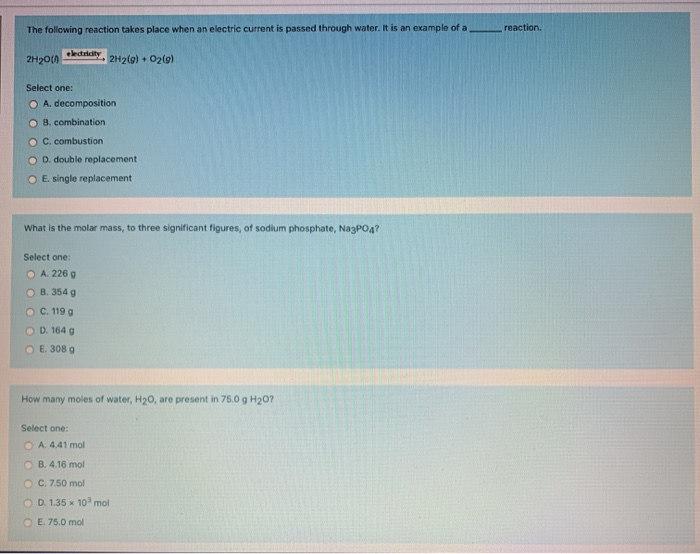The following reaction takes place when an electric current is passed through water. It is an example of a reaction 2H200 dolary, 2H2(g) + O2(g) Select one: A decomposition O 8. combination OC. combustion O D. double replacement E single replacement What is the molar mass, to three significant figures, of sodium phosphate, NagPO4? Select one: O A. 2269 8.354 g O C. 1190 D. 1640 E. 308 g How many moles of water, H20, are present in 75.0 g H20? Select one: A. 4.41 mol OB. 4.16 mol C. 7.50 mol D. 1.35 x 10 mol E. 75.0 mol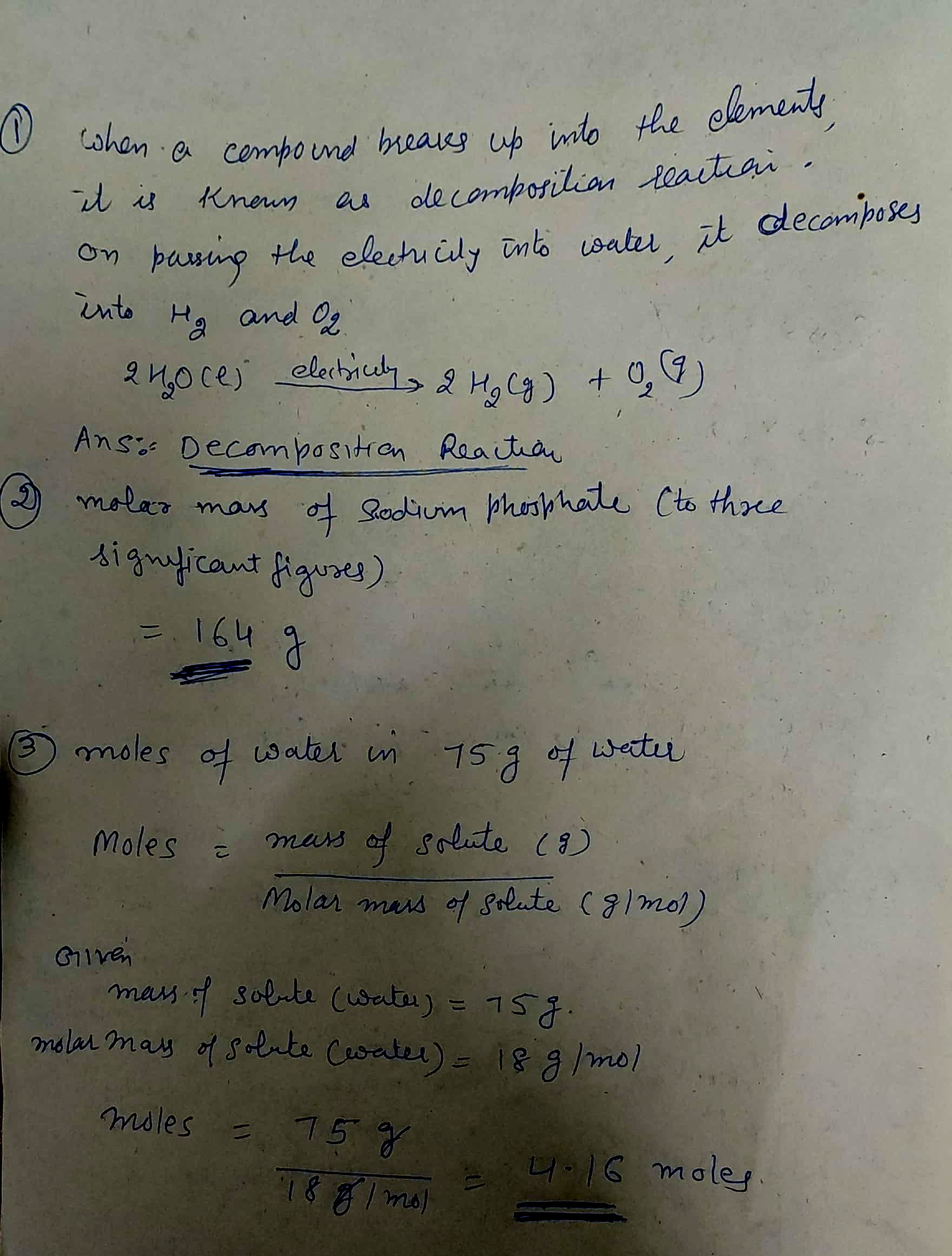#### Earn Coins

Coins can be redeemed for fabulous gifts.

Similar Homework Help Questions
• ### AH = 44.0 kJ/mol AS = 0.119 kJ/mol K The following reaction takes place at 120°C:...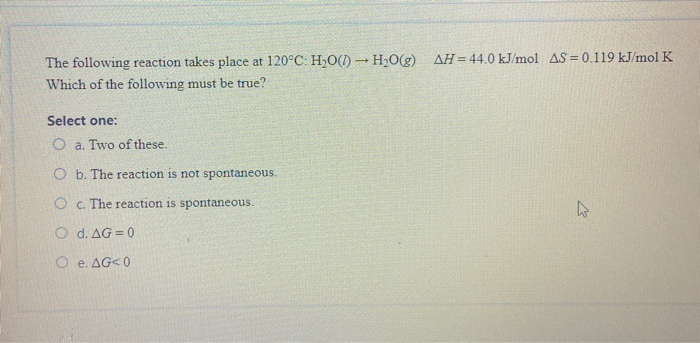AH = 44.0 kJ/mol AS = 0.119 kJ/mol K The following reaction takes place at 120°C: H20(1) - H2O(g) Which of the following must be true? Select one: O a. Two of these O b. The reaction is not spontaneous O c The reaction is spontaneous. O d. AG = 0 e. AGO

• ### The following reaction takes place when an electric current is passed through

13. The following reaction takes place when an electric current is passed through 2 H2O → O2 + 2 H2O It is an example of a .... .....reaction.  14. What is oxidized and what is reduced in the following reaction? 2 Al(s) + 3 Br2(i) → 2 AlBr3

• ### 49. Calculate the mass of water produced when 625 of methane, CH4, reacts with an excess...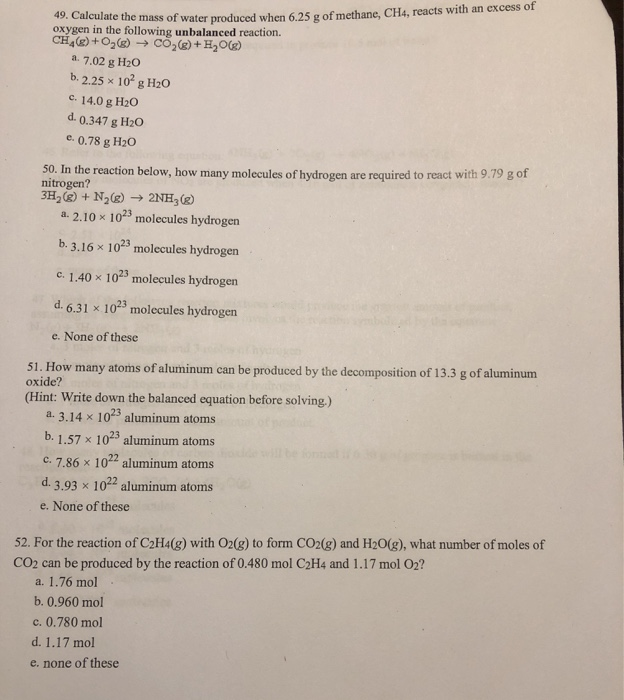49. Calculate the mass of water produced when 625 of methane, CH4, reacts with an excess of oxygen in the following unbalanced reaction. CH4%)+18) + CO2g)+H2Og) a. 7.02 g H20 b. 2.25 x 10² g H20 c. 14.0 g H20 d. 0.347 g H20 c. 0.78 g H20 50. In the reaction below, how many molecules of hydrogen are required to react with 9.79 g of nitrogen? 3H,@) + N2) 2NH3) 1.2.10 x 1023 molecules hydrogen b. 3.16 x 1023...

• ### 10. What is a chemical reaction? A) when a liquid undergoes a phase change and produces...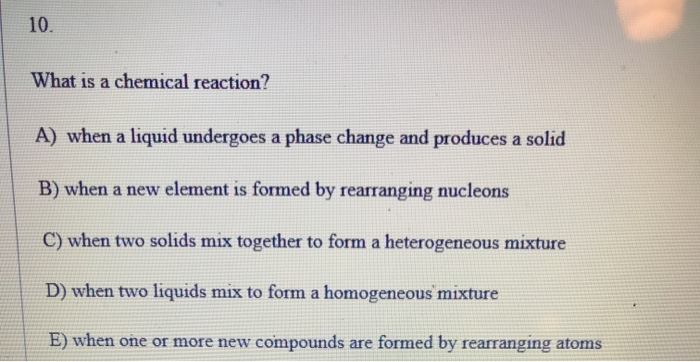10. What is a chemical reaction? A) when a liquid undergoes a phase change and produces a solid B) when a new element is formed by rearranging nucleons C) when two solids mix together to form a heterogeneous mixture D) when two liquids mix to form a homogeneous mixture E) when one or more new compounds are formed by rearranging atoms 17. Identify the reaction type: Al2O3(s) → O2(g) + Al(s) A) combustion B) combination C) decomposition D) single replacement...

• ### 12) 12) Choose the best classification of the reaction represented by the following equation: 2Fe3 (ag)+...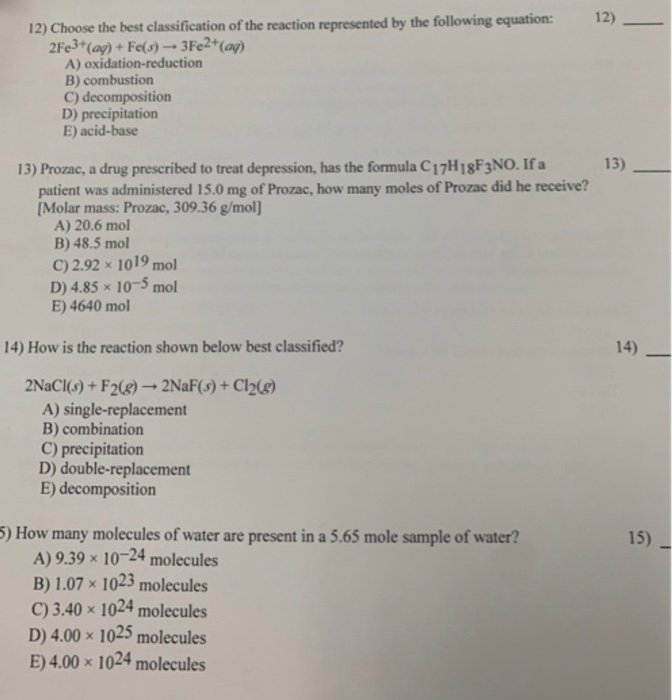12) 12) Choose the best classification of the reaction represented by the following equation: 2Fe3 (ag)+ Fe(s)3Fe2+(ag) A) oxidation-reduction B) combustion C) decomposition D) precipitation E) acid-base 13) 13) Prozac, a drug prescribed to treat depression, has the formula C17H18F3NO. If a patient was administered 15.0 mg of Prozac, how many moles of Prozac did he receive? [Molar mass: Prozac, 309.36 g/mol] A) 20.6 mol B) 48.5 mol C) 2.92 x 1019 mol D) 4.85x 10-5mol E) 4640 mol 14)...

• ### QUESTION5 When sodium is added to water, the following reaction takes place: Na(s)H20NaOH(aq) 1/2H2(g) What can...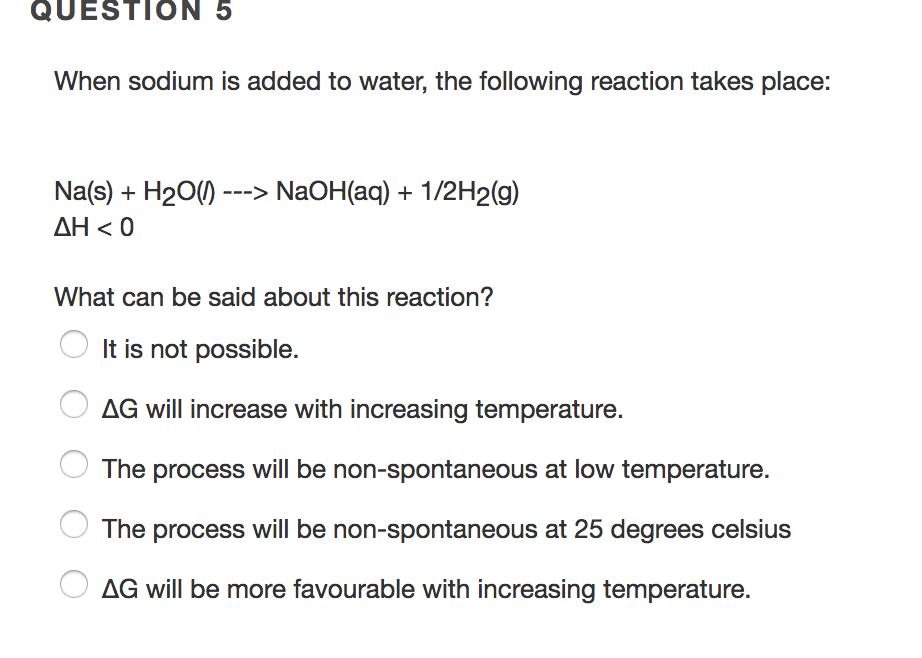QUESTION5 When sodium is added to water, the following reaction takes place: Na(s)H20NaOH(aq) 1/2H2(g) What can be said about this reaction? It is not possible. AG will increase with increasing temperature. The process will be non-spontaneous at low temperature. The process will be non-spontaneous at 25 degrees celsius AG will be more favourable with increasing temperature.

• ### In the following reaction, how many grams of H20 are produced if 4.29 g of NzH4...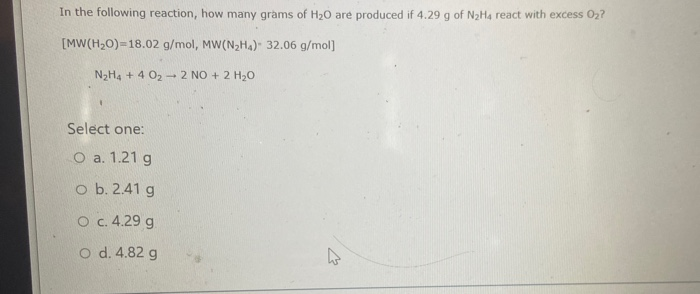In the following reaction, how many grams of H20 are produced if 4.29 g of NzH4 react with excess 02? [MW(H20)=18.02 g/mol, MW(N2Ha). 32.06 g/mol] N H4 + 4 02 - 2 NO + 2 H2O Select one: O a. 1.21 g ob. 2.41g O c.4.29 g o d. 4.82 g

• ### in 19 The following reaction takes place at 120°C-H 0(1) - H 0(g) Which of the...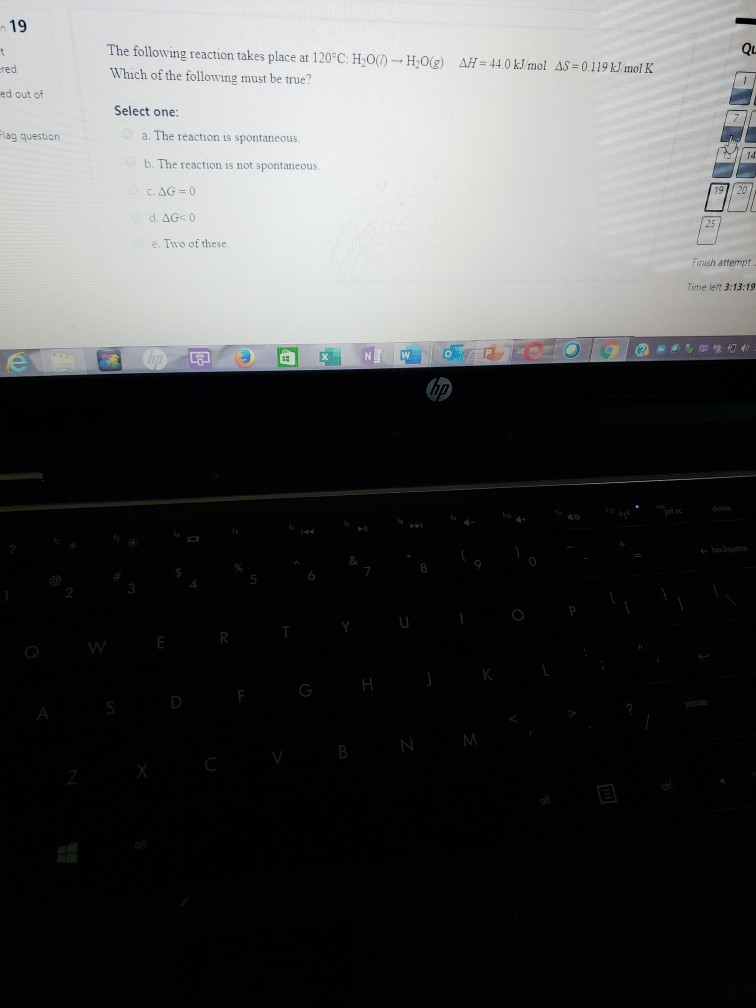in 19 The following reaction takes place at 120°C-H 0(1) - H 0(g) Which of the following must be true? AH = 44.0 kJ mol AS = 0.119 kJ mol K ered ed out of Ein Select one: a. The reaction is spontaneous Flag question b. The reaction is not spontaneous CAG=0 d. AGO e. Two of these Finish attempt. Time left 3:13:19 e lp @ NI Wololol **** 2x Cy BN м.

• ### 1) 1) What is the stoichiometric coefficient for water when the following equation is balanced using...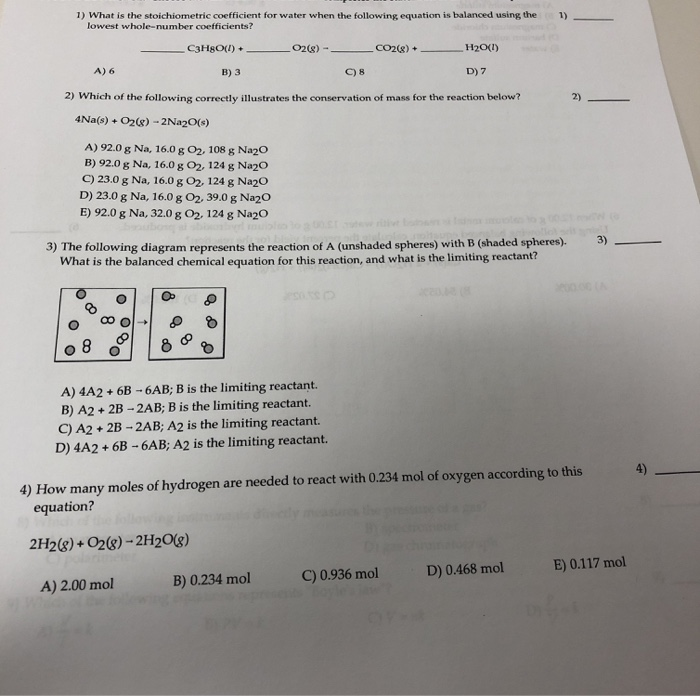1) 1) What is the stoichiometric coefficient for water when the following equation is balanced using the lowest whole-number coefficients? _C3HgO(l) + _02(8) - — CO2(g) + ___ _H2O(1) A) 6 B) 3 098 D) 7 2) Which of the following correctly illustrates the conservation of mass for the reaction below? 2) 4Na(s) + O2(g) - 2Na2O(s) A) 92.0 g Na, 16.0 g O2, 108 g Na2O B) 92.0 g Na, 16.0 g 02, 124 g Na2O C) 23.0 g...

• ### 10) Consider the following balanced e following balanced reaction. How many grams of water are required...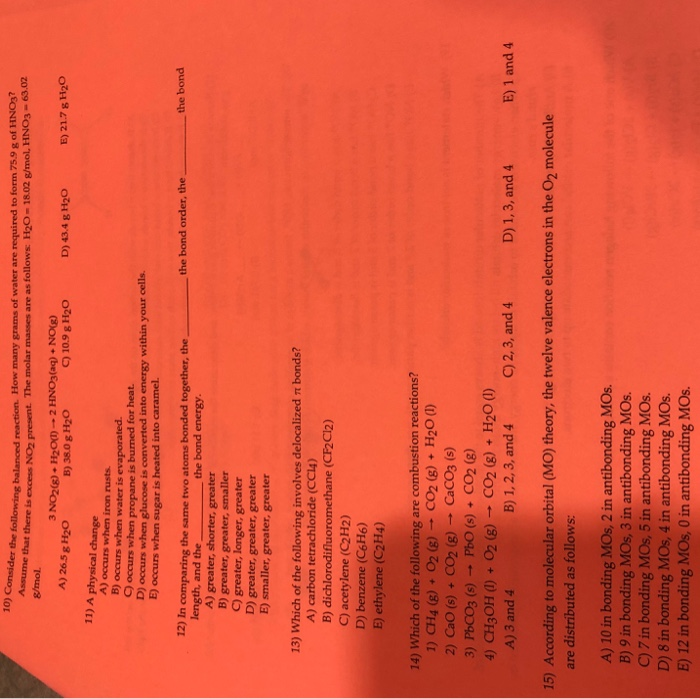10) Consider the following balanced e following balanced reaction. How many grams of water are required to form 75.9 g of HNO3? that there is excess NO present. The molar masses are as follows: H2O - 18.02 g/mol, HNO3 - 63.02 g/mol. A) 26.5 g H20 3 NO2(g) + H2O(1) -- 2 HNO3(aq) + NO(g) B ) 38.0 g H20 C) 10.9 g H20 D) 43.4 g H20 E) 21.7 g H20 11) A physical change A) occurs when iron...